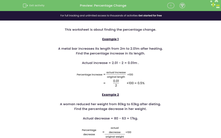# Calculate the Percentage Change

In this worksheet, students will work out percentage changes.Key stage:  KS 3

Curriculum topic:   Number

Curriculum subtopic:   Interpret Fractions/Percentages as Operators

Difficulty level:#### Worksheet Overview

This activity is about finding percentage change.

Example 1

A metal bar increases its length from 2 m to 2.01 m after heating.

Find the percentage increase in its length.

Actual increase = 2.01 - 2 = 0.01 m

Percentage increase = (actual increase ÷ original length) x 100 = (0.01 ÷ 2) x 100 = 0.5%

Example 2

A woman reduced her weight from 80 kg to 63 kg after dieting.

Find the percentage decrease in her weight.

Actual decrease = 80 - 63 = 17 kg.

Percentage decrease = (actual decrease ÷ original weight) x 100 = (17 ÷ 80) x 100 = 21.25Just remember the formula for percentage change = (actual change ÷ original) x 100

Let's try some questions now.

### What is EdPlace?

We're your National Curriculum aligned online education content provider helping each child succeed in English, maths and science from year 1 to GCSE. With an EdPlace account you’ll be able to track and measure progress, helping each child achieve their best. We build confidence and attainment by personalising each child’s learning at a level that suits them.

Get started